# ML Wiki

## Workflow Patterns

Workflow patterns help to express the constructions common to workflows

## Basic Control Flow Patterns

### Sequence

First task $A$, then task $B$ :

•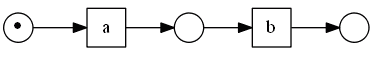### Parallel Split

Tasks $b$ and $c$ are processed in parallel 

•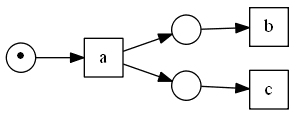### Synchronization

Do $d$ only after both $b$ and $c$ are completed 

• this is a synchronization between two parallel processes
•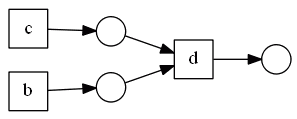### Exclusive Choice

after $a$, do $b$ or $c$ 

•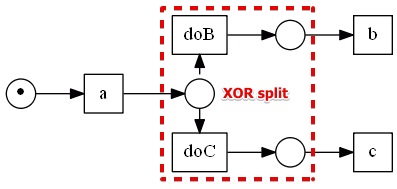### Simple Merge

perform $d$ after $b$ or $c$ finishes 

•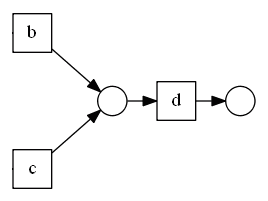## Termination Patterns

• Implicit Termination